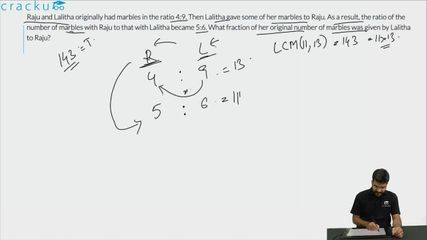Question 83

# Raju and Lalitha originally had marbles in the ratio 4:9. Then Lalitha gave some of her marbles to Raju. As a result, the ratio of the number of marbles with Raju to that with Lalitha became 5:6. What fraction of her original number of marbles was given by Lalitha to Raju?

Solution

Let the number of marbles with Raju and Lalitha initially be 4x and 9x.
Let the number of marbles that Lalitha gave to Raju be a.

It has been given that (4x+a)/(9x-a) = 5/6
24x + 6a = 45x - 5a
11a = 21x
a/x = 21/11

Fraction of original marbles given to Raju by Lalitha = a/9x (Since Lalitha had 9x marbles initially).
a/9x = 21/99
= 7/33.

Therefore, option D is the right answer.

### View Video Solution